# GRE Quantitative Reasoning - Plane Geometry: Tutoring Solution Chapter Exam

Exam Instructions:

Choose your answers to the questions and click 'Next' to see the next set of questions. You can skip questions if you would like and come back to them later with the yellow "Go To First Skipped Question" button. When you have completed the practice exam, a green submit button will appear. Click it to see your results. Good luck!

### Page 1

#### Question 2 2. The pictured shape is a: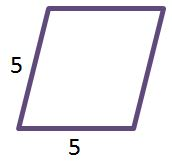### Page 2

#### Question 6 6. Given the figure below, Angle 3 = 140y and Angle 5 = 80y + 70. Determine the value of y.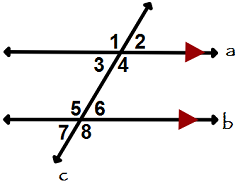#### Question 8 8. What is the area of ABCDE, as shown in the diagram?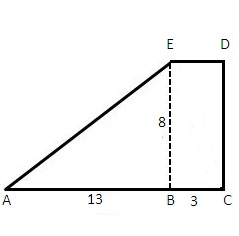### Page 3

#### Question 11 11. Which of the following is true?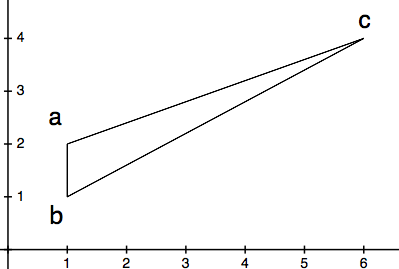#### Question 12 12. In triangle GBN, Angle G is seven more than three times a number, Angle B is five more than four times the number, and Angle N is twelve less than five times the number. Solve for the measure of Angle G.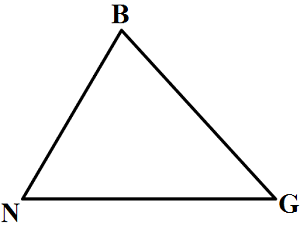#### Question 13 13. The pictured shape is a: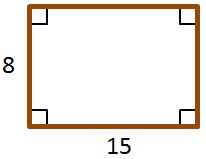#### Question 14 14. Given the triangle ABC, solve for the value of y.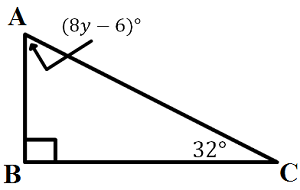### Page 4

#### Question 16 16. Josh claims that these triangles are similar by SSS. Do you agree or disagree with Josh?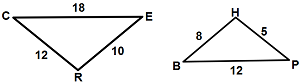#### Question 17 17. What is the area of the right triangle shown below?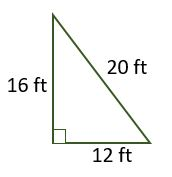#### Question 18 18. Is triangle ABC similar to triangle DEF?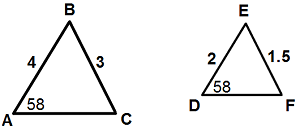### Page 5

#### Question 21 21. Triangle HYV and triangle AYB are similar by the AA similarity theorem. What is the value of x?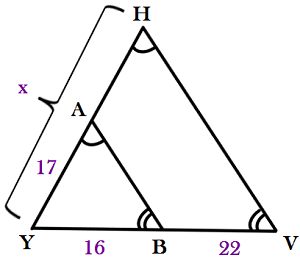#### Question 23 23. Mary claims that the triangles below are similar by AA. Do you agree or disagree with Mary?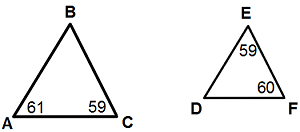#### Question 25 25. What is the area of the pictured rectangle?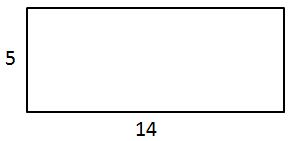### Page 6

#### Question 26 26. Given the triangle PQE, calculate the measure of Angle Q.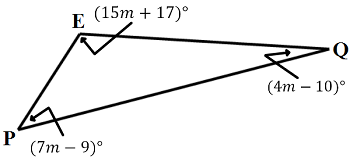#### GRE Quantitative Reasoning - Plane Geometry: Tutoring Solution Chapter Exam Instructions

Choose your answers to the questions and click 'Next' to see the next set of questions. You can skip questions if you would like and come back to them later with the yellow "Go To First Skipped Question" button. When you have completed the practice exam, a green submit button will appear. Click it to see your results. Good luck!

Support